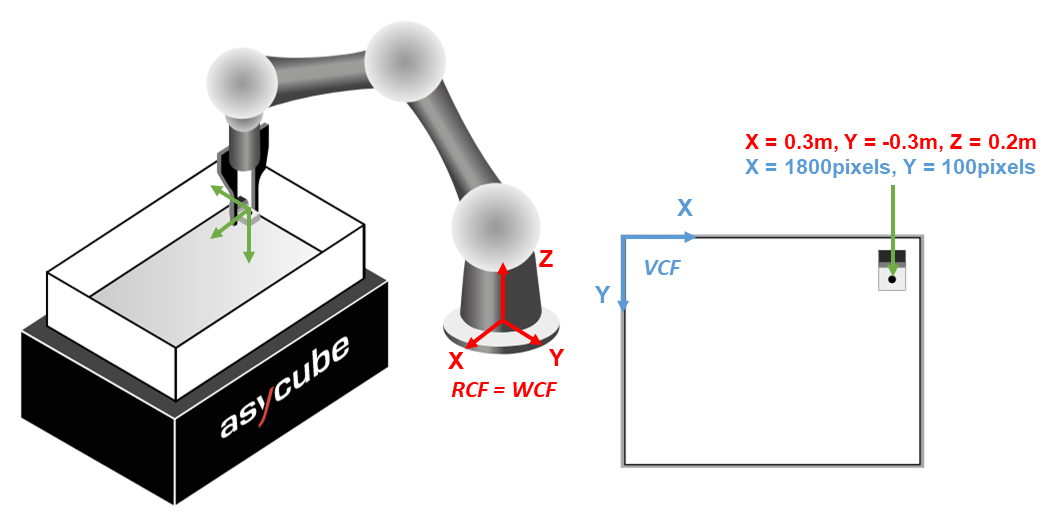Warning

You are reading an old version of this documentation. If you want up-to-date information, please have a look at 3.3 .

# What is hand-eye calibration?

The question is to find the relative position and orientation between a rigidly mounted camera and the the last joint of the robot gripper. The target of the hand-eye calibration is to transform the detected parts X,Y coordinates from the vision coordinate frame (VCF) to the robot coordinate frame (RCF).

After hand-eye calibration, EYE+ will be able to directly send the robot coordinates (in the robot frame) after each image acquisition.Fig. 228 Hand-eye calibration representation; RCF: red coordinate system; VCF: blue coordinate system.

## How is it solved?

The mathematical problem takes the form of the affine transformation:

$X_r = A X_C + B$

where $$X_r$$ are coordinates in $$(x,y)$$ in the robot frame and $$X_c$$ are coordinates in $$(x,y)$$ in the camera frame. The transformation matrices $$A$$ and $$B$$ are deduced from the hand-eye calibration. Thanks to these, the coordinates in the robot system can be deduced from the coordinates in the vision system.

Warning

The difference in height between the two frames is not handled by EYE+. The hand-eye calibration is only performing coordinate transformation of the $$(x,y)$$ positions. Your robot must be aware of the difference in height between its frame and the plate, as well as the difference in height between the plate and the height of the part.

## Calibration accuracy

The calibration accuracy displayed in step 6 is the root mean square of the reprojection error of the four points. The reprojections are the inverse transformations of the 4 robot points projected in the vision frame.

The smaller the calibration accuracy value, the better the calibration.